# fast.ai lesson07

Oct 18, 2019 · 37 mins read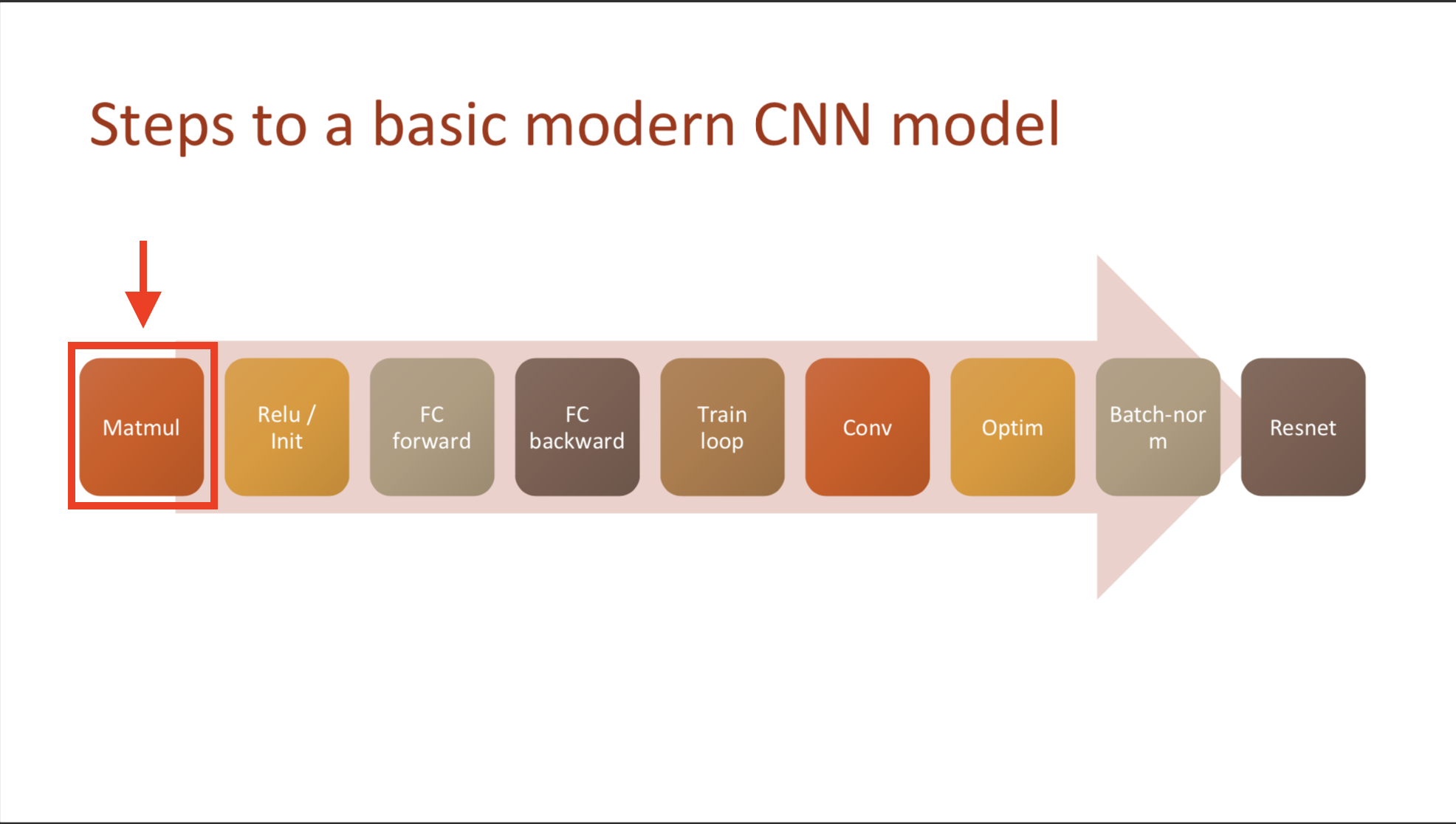” Lecture 08 - Deep Learning From Foundations-part2 “

### Homework

• Review concepts 16 concepts from Course 1 (lessons 1 - 7) (1) Affine Functions & non-linearities; 2) Parameters & activations; 3) Random initialization & transfer learning; 4) SGD, Momentum, Adam; 5) Convolutions; Batch-norm; 6) Dropout; 7) Data augmentation; 8) Weight decay; 9) Res/dense blocks; 10) Image classification and regression; 11)Embeddings; 12) Continuous & Categorical variables; 13) Collaborative filtering; 14) Language models; 15) NLP classification; 16) Segmentation; U-net; GANS)

## What is going on in this course?

### What is ‘from foundations’?

1) Recreate fast.ai and Pytorch

2) using pure python

Overfit : validation error getting worse training loss < validation loss

• Know the name of the symbol you use

find in this page if you don’t know the symbol that you are using or just draw it here (run by ML!)

### Steps to a basic modern CNN model

1) Matrix multiplication -> 2) Relu/Initialization -> 3) Fully-connected Forward -> 4) Fully-connected Backward -> 5) Train loop -> 6) Convolution-> 7) Optimization -> 8) Batchnormalization -> 9) Resnet

## Library development using jupyter notebook

what is assers?

### jupyter notebook certainly can make module

• There will be #export tag that Howard (and we) want to extract
• special notebook2script.py will detect sign of #expert and convert following into python module
• and test it

test\_eq(TEST,'test')test\_eq(TEST,'test1')

• what is run_notebook.py?
• when you want to test your module in command line interface

 !python run\_notebook.py 01_matmul.ipynb

• Is there any difference between 1) and 2)?

1) test -> test01 2) test01 -> test

#TODO I don’t know yet

• look into run_notebook.py, package fire Jeremy used. What is that?

read and run the code in a notebook, and in the process, Jeremy made Python Fire library called!shockingly, fire takes any kind of function and converts into CLI command.

fire library was released by Google open source, Thursday, March 2, 2017

• Get data

• pytorch and numpy are pretty much same.
• variable c explains how many pixels there are in in MNIST, 28 pixels
• PyTorch’s view() method: torch function that manipulating tensor, and squeeze() in torch & mathmatical operation similar function
• Rao & McMahan said usually this functions result in feature vector.
• In part 1, you can use view function several times.

• Initial python model

• Which is Linear, like $Xw$(weight)$+a$(bias) $= Y$

• If you don’t know hou to multiple matrix, refer this site matmul visulization site

• How many time spends if we we use pure python
• function matmul, typical matrix multiplication function, takes about 1 second for calculating 1 single train data! (maybe assumed stochastic, 5 data points in validation)

• it takes about 11.36 hours to update parameters even single layer and 1 iteration! (if that was my computer, it would be 14 hours..)🤪

• THIS is why we need to consider ‘time’&’space’

This is kinda slow - what if we could speed it up by 50,000 times? Let’s try!

## Elementwise ops

### How can we make python faster?

• If we want to calculate faster, then do remove pythonic calcuation, by passing its computation down to something that is written something other than python, like pytorch.
• According to PyTorch doc it uses C++ (via ATen), so we are going to implement that function with python.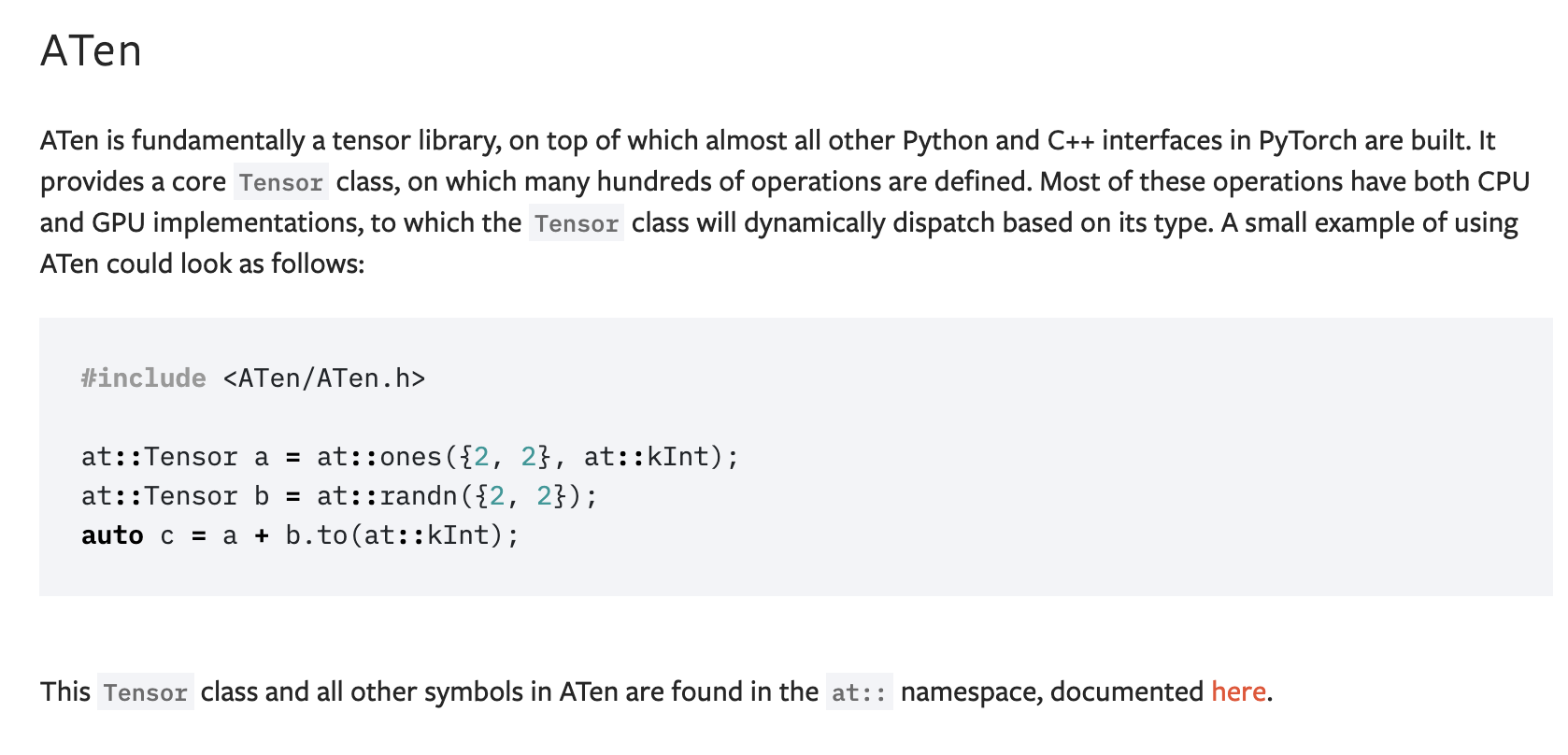#### What is element wise operation?

• items makes a pair, operate corresponding component

## Resources

Section02

### Time comparison with pure Python

> 3194.95 times faster

• Einstein summation
> 16090.91 times faster

• Pytorch’s operator
> 49166.67 times faster

### 1. Elementwise op

#### 1.1 Frobenius norm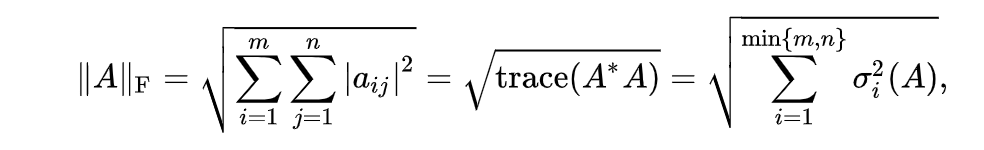• above converted into
(m*m).sum().sqrt()

• Plus, don’t suffer from mathmatical symbols. He also copy and paste that equations from wikipedia.

### 2. Elementwise Matmul

• What is the meaning of elementwise?
• We do not calculate each component. But all of the component at once. Because, length of column of A and row of B are fixed.

• How much time we saved?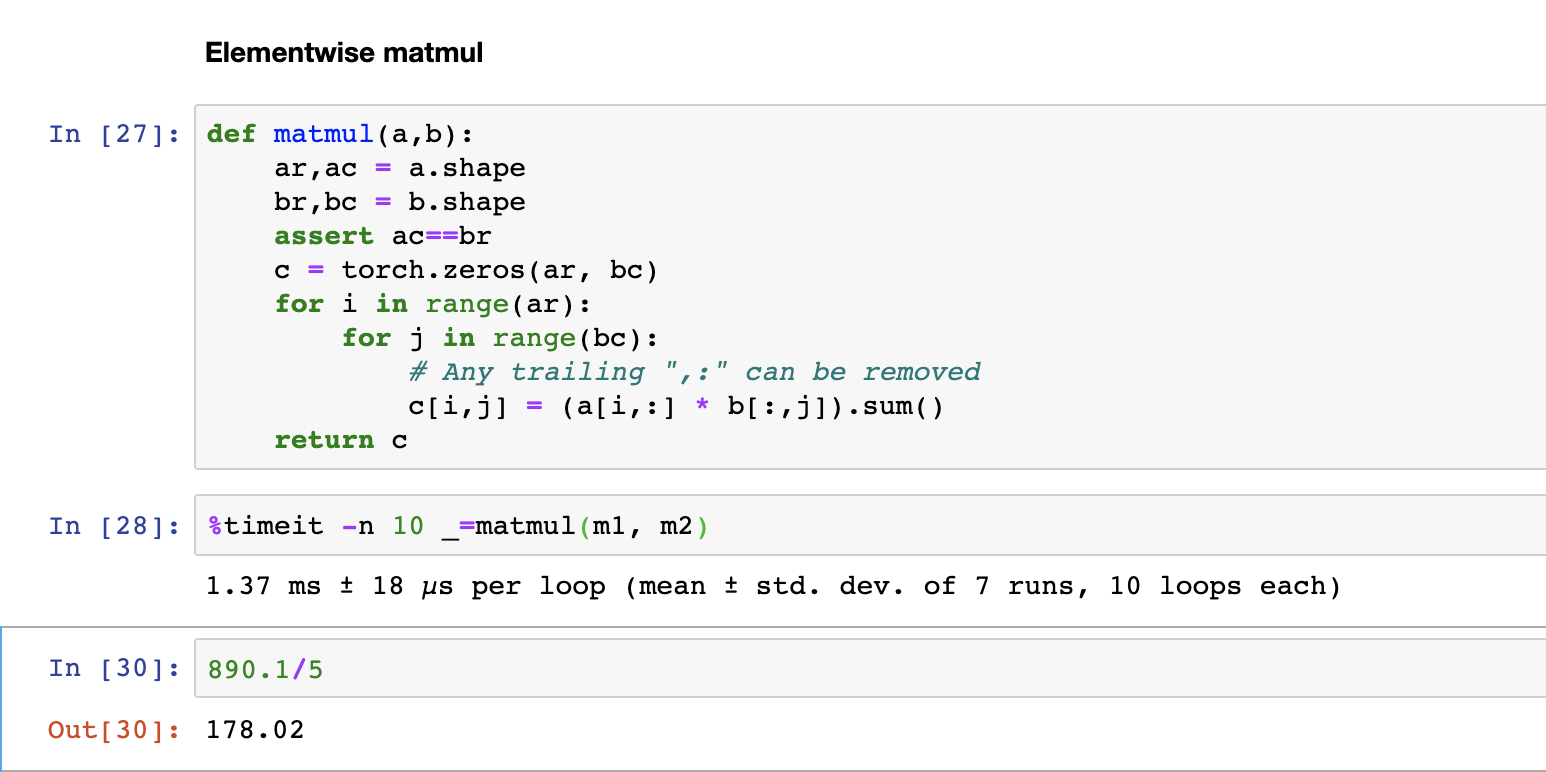• So now that takes 1.37ms. We have removed one line of code and it is a 178 times faster…

#TODO I don’t know where the 5 from. but keep it. Maybe this is related with frobenius norm…? as a result, the code before

for k in range(ac):
c[i,j] += a[i,k] + b[k,j]


the code after

c[i,j] = (a[i,:] * b[:,j]).sum()


To compare it (result betweet original and adjusted version) we use not test_eq but other function. The reason for this is that due to rounding errors from math operations, matrices may not be exactly the same. As a result, we want a function that will “is a equal to b within some tolerance

#export
def near(a,b):

def test_near(a,b):
test(a,b,near)

test_near(t1, matmul(m1, m2))


• Now, we will use the broadcasting and remove
c[i,j] = (a[i,:] * b[:,j]).sum()

• How it works?
>>> a=tensor([[10,10,10],
[20,20,20],
[30,30,30]])
>>> b=tensor([1,2,3,])

>>> a,b

(tensor([[10, 10, 10],
[20, 20, 20],
[30, 30, 30]]),
tensor([1, 2, 3]))


>>> a+b

tensor([[11, 12, 13],
[21, 22, 23],
[31, 32, 33]])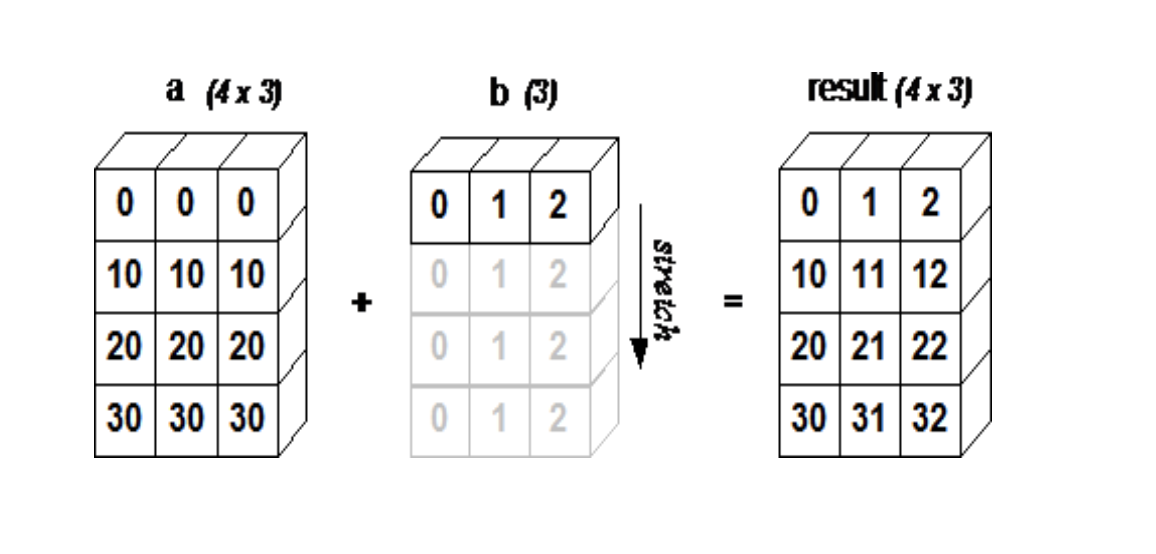• <Figure 2> demonstrated how array b is broadcasting(or copied but not occupy memory) to compatible with a. Refered from numpy_tutorial
• there is no loop, but it seems there is exactly the loop.

• This is not from jeremy (actually after a moment he cover it) but i wondered How to broadcast an array by columns?

c=tensor([,,])
a+c


tensor([[11, 11, 11], [22, 22, 22], [33, 33, 33]])s

• What is tensor.stride()?
help(t.stride)


Help on built-in function stride:
stride(…) method of torch.
Tensor instance
stride(dim) -> tuple or int
Returns the stride of :attr:’self’ tensor.
Stride is the jump necessary to go from one element to the next one in the specified dimension :attr:’dim’.
A tuple of all strides is returned when no argument is passed in.
Otherwise, an integer value is returned as the stride in the particular dimension :attr:’dim’.

Args: dim (int, optional): the desired dimension in which stride is required Example::*

x = torch.tensor([[1, 2, 3, 4, 5], [6, 7, 8, 9, 10]])
x.stride()
>>> (5, 1)
x.stride(0)
>>> 5
x.stride(-1)
>>> 1

• unsqueeze & None index

• We can manipulate rank of tensor
• Special value ‘None’, which means please squeeze a new axis here
c = torch.tensor([10,20,30])
c[None,:]

• in c, squeeze a new axis in here please.

for i in range(ar):
#   c[i,j] = (a[i,:]).          *[:,j].sum() #previous
c[i]   = (a[i].unsqueeze(-1) * b).sum(dim=0)

• And Using None also (As howard teached)
c[i]   = (a[i  ].unsqueeze(-1) * b).sum(dim=0) #howard
c[i]   = (a[i][:,None] * b).sum(dim=0) # using None
c[i]   = (a[i,:,None]*b).sum(dim=0)


⭐️Tips🌟
1) Anytime there’s a trailinng(final) colon in numpy or pytorch you can delete it ex) c[i, :] = c [i] 2) any number of colon commas at the start, you can switch it with the single elipsis. ex) c[:,:,:,:,i] = c […,i]

• What if we tensor.size([1,3]) * tensor.size([3,1])?
torch.Size([3, 3])

• What is scale????
• What if they are one array is times of the other array?
ex) Image : 256 x 256 x 3
Scale : 128 x 256 x 3
Result: ?

• Why I did not inserted axis via None, but happened broadcasting?
>>> c * c[:,None]
tensor([[100., 200., 300.],
[200., 400., 600.],
[300., 600., 900.]])


maybe it broadcast cz following array has 3 rows

as same principle, no matter what nature shape was, if we do the operation tensor broadcasts to the other.

>>> c==c[None]
tensor([[True, True, True]])

>>> c[None]==c[None,:]
tensor([[True, True, True]])

>>>c[None,:]==c
tensor([[True, True, True]])


### 3. Einstein summation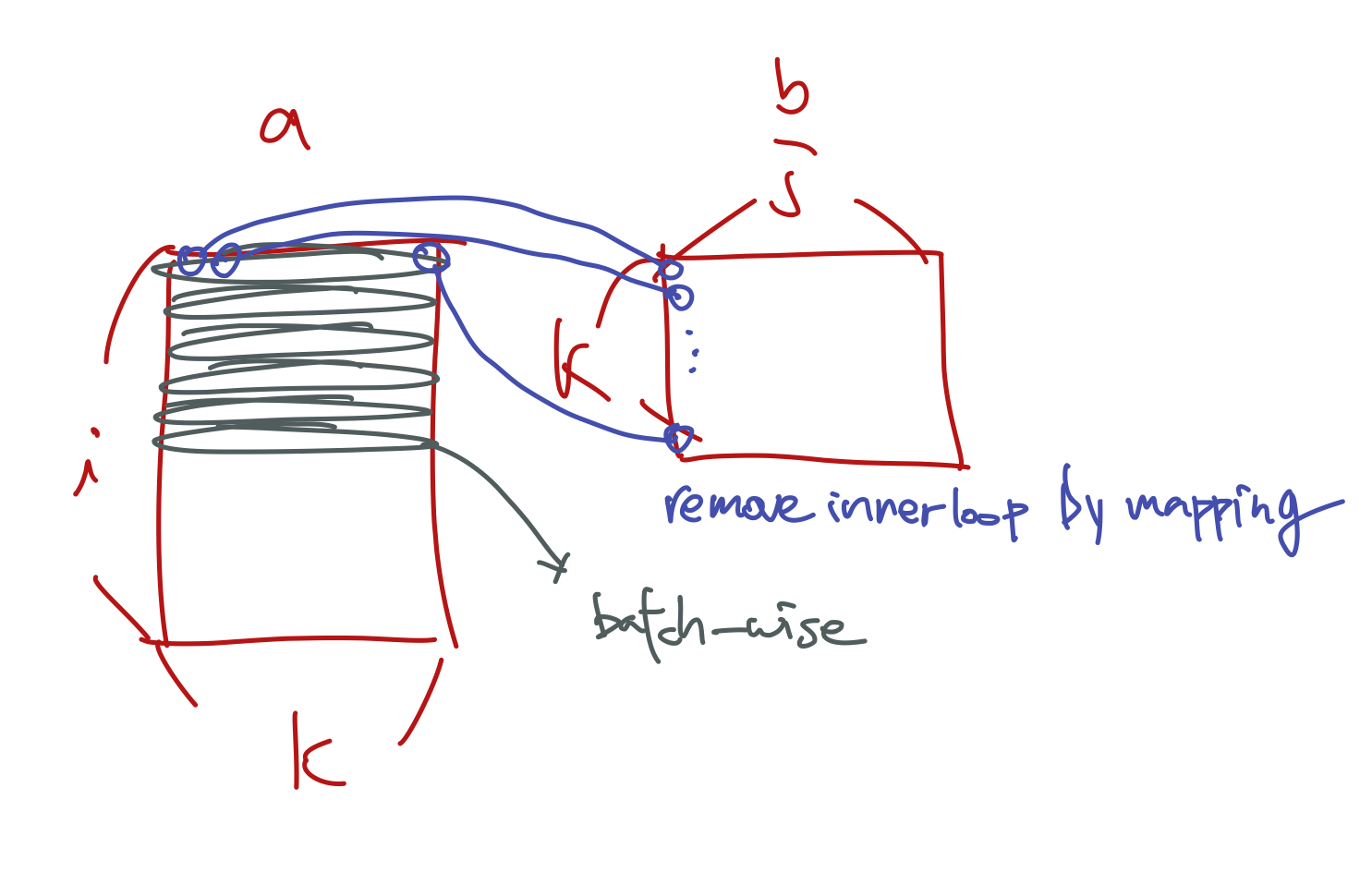• Creates batch-wise, remove inner most loop, and replaced it with an elementwise product a.k.a
c[i,j] += a[i,k] * b[k,j]


inner most loop

c[i,j] = (a[i,:] * b[:,j]).sum()


elementwise product

• Because K is repeated so we do a dot product. And it is torch.

Usage of einsum() 1) transpose 2) diagnalisation tracing 3) batch-wise (matmul)

• einstein summation notation
def matmul(a,b): return torch.einsum('ik,kj->ij', a, b)


so after all, we are now 16000 times faster than Python.

### 4. Pytorch op

49166.67 times faster than pure python

And we will use this matrix multiplication in Fully Connect forward, with some initialized parameters and ReLU.

But before that, we need initialized parameters and ReLU,

## Resources

• Frobenius Norm Review
• Refer colab! (I totally confused with extension of arrays)
• torch.allclose Review
• np.einsum Review

section03

### 1. The forward and backward passes

#### 1.1 Normalization

train_mean,train_std = x_train.mean(),x_train.std()
>>> train_mean,train_std
(tensor(0.1304), tensor(0.3073))


Remember!

• Dataset, which is x_train, mean and standard deviation is not 0&1. But we need them to be which means we should substract means and divide data by std.
• You should not standarlize validation set because training set and validation set should be aparted.
• after normalize, mean is close to zero, and standard deviation is close to 1.

#### 1.2 Variable definition

• n,m: size of the training set
• c: the number of activations we need in our model

### 2. Foundation Version

#### 2.1 Basic architecture

• Our model has one hidden layer, output to have 10 activations, used in cross entropy.
• But in process of building architecture, we will use mean square error, output to have 1 activations and lator change it to cross entropy

• number of hidden unit; 50

see below pic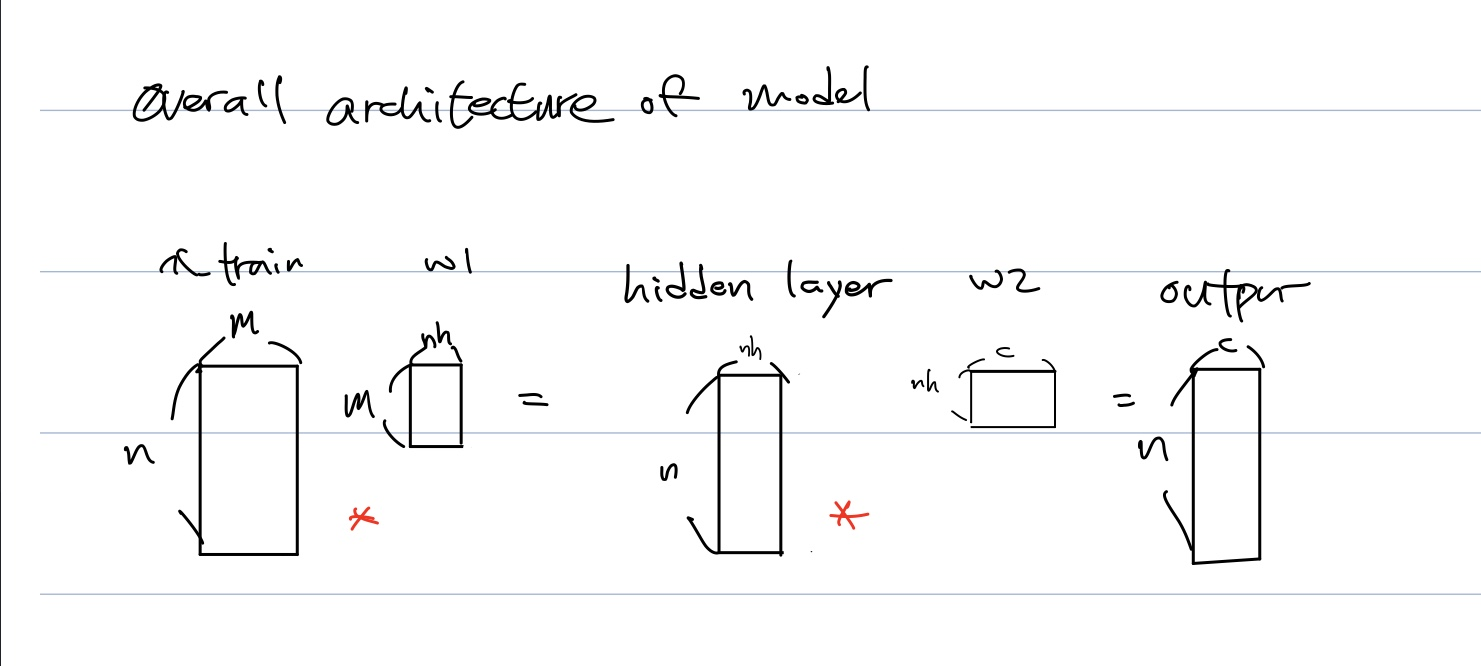• We want to make w1&w2 mean and std be 0&1.
• why initializating and make mean zero and std one is important?
• paper highlighting importance of normalisation - training 10,000 layer network without regularisation1
##### 2.1.1 simplified kaiming init

Q: Why we did init, normalize with only validation data? Because we can not handle and get statistics from each value of x_valid?{: style=”color:red; font-size: 130%; text-align: center;”}

w1 = torch.randn(m,nh)
b1 = torch.zeros(nh)
t = lin(x_valid, w1, b1) # hidden

>>> t.mean(), t.std()

((tensor(2.3191), tensor(27.0303))


In output(second) layer,

w2 = torch.randn(nh,1)
b2 = torch.zeros(1)
t2 = lin(t, w2, b2) # output

>>> t2.mean(), t2.std()

(tensor(-58.2665), tensor(170.9717))

• which is terribly far from normalzed value.

• But if we apply simplified kaiming init

w1 = torch.randn(m,nh)/math.sqrt(m); b1 = torch.zeros(nh)
w2 = torch.randn(nh,1)/math.sqrt(nh); b2 = torch.zeros(1)
t = lin(x_valid, w1, b1)
t.mean(),t.std()
>>> (tensor(-0.0516), tensor(0.9354))

• But, actually, we use activations not only linear function
• After applying activations relu at linear layer, mean and deviation became 0.5.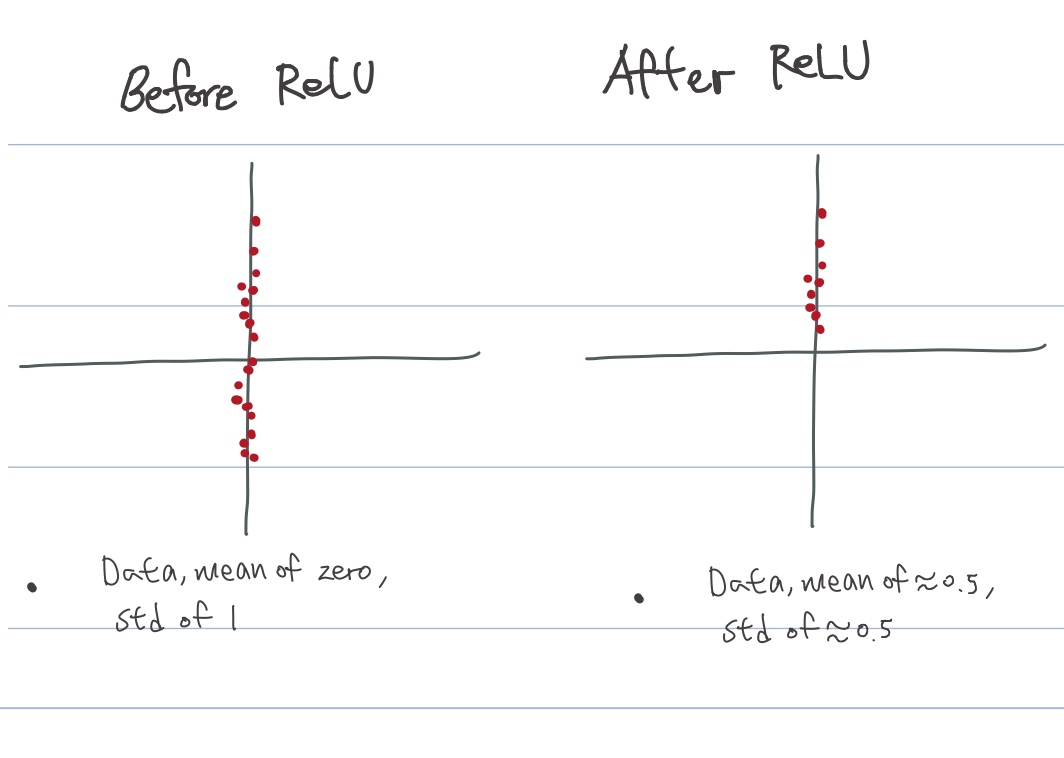##### 2.1.2 Glorrot initialization
• Gaussian(, bell shaped, normal distributions) is not trained very well.
• How to initialize neural nets?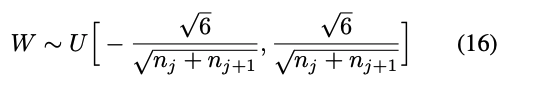with $n_i$ the size of layer $n$, the number of filters $i$.

• But there is No acount for import of ReLU
• If we got 1000 layers, vanishing gradients problem emerges
##### 2.1.3 Kaiming initializating
• Kaiming He, explained here
• rectifier: rectified linear unit
• rectifier network: neural network with rectifier linear units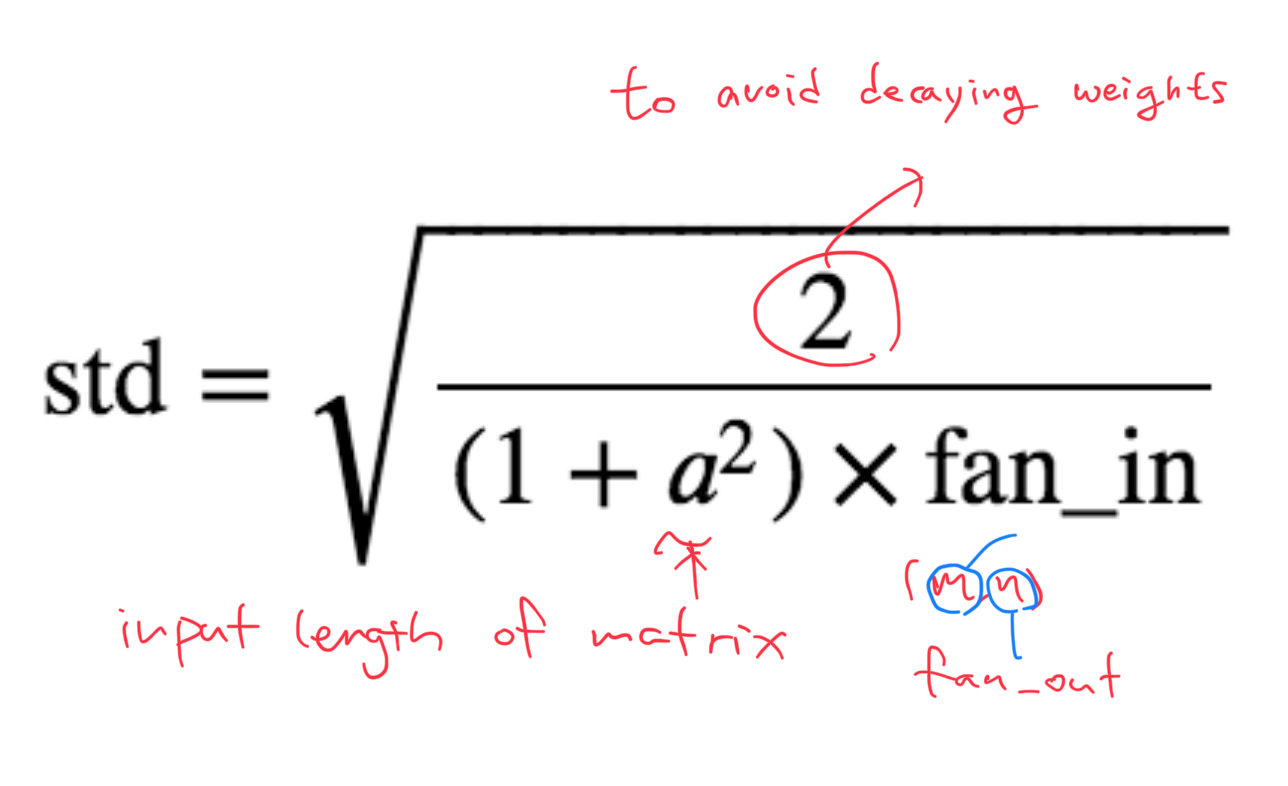• This is kaiming init, and why suddenly replace one to two on a top?
• But it doesn’t give very nice mean tough.
##### 2.1.4 Pytorch package
• Why fan_out?
• according to pytorch documentation,

choosing 'fan_in' preserves the magnitude of the variance of the wights in the forward pass.

choosing 'fan_out' preserves the magnitues in the backward pass(, which means matmul; with transposed matrix)

➡️ in the other words, torch use fan_out cz pytorch transpose in linear transformaton.

• What about CNN in Pytorch?

I tried

torch.nn.Conv2d.conv2d_forward??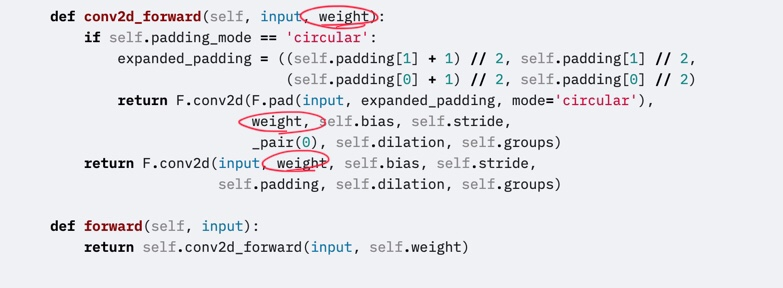Jeremy digged into using

torch.nn.modules.conv._ConvNd.reset_parameters??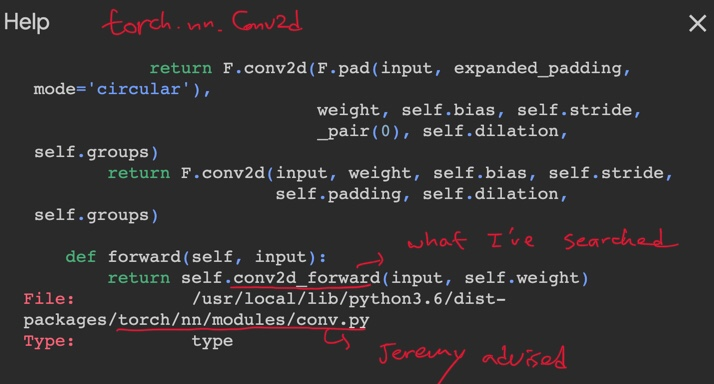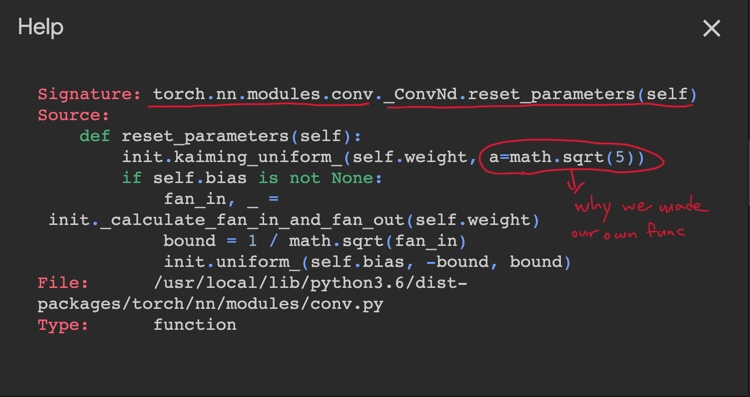2

• in Pytorch, it doesn’t seem to be implemented kaiming init in right formula. so we should use our own operation.
• But actually, this has been discussed in Pytorch community before.3 4
• Jeremy said it enhanced variance also, so I sampled 100 times and counted better results.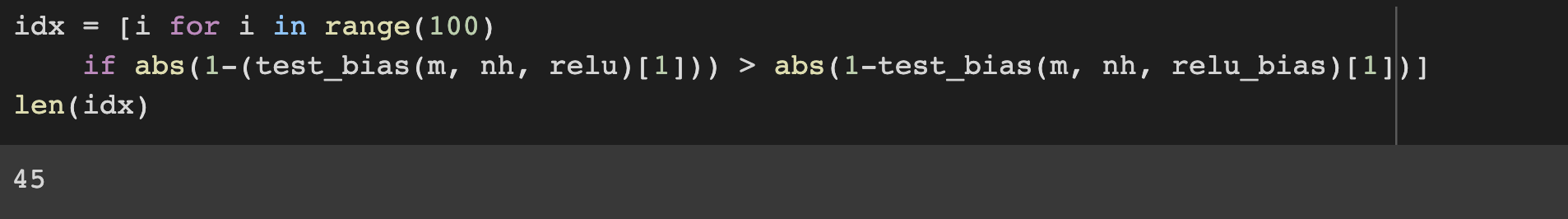• To make sure the shape seems sensible. check with assert. (remember we will replace 1 to 10 in cross entropy)
assert model(x_valid).shape==torch.Size([x_valid.shape,1])
>>> model(x_valid).shape
(10000, 1)

• We have made Relu, init, linear, it seems we can forward pass
• code we need for basic architecture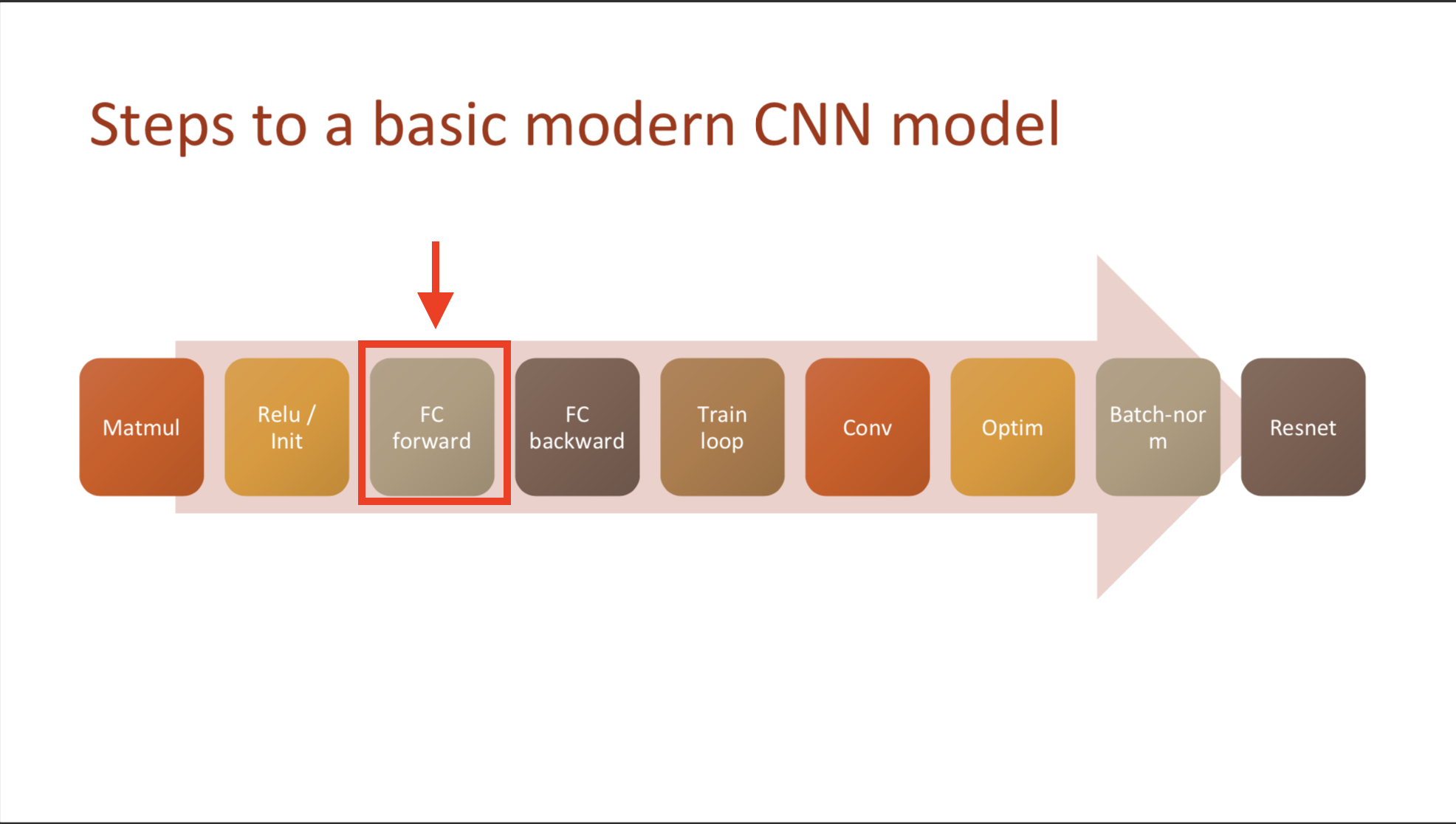nh = 50
def lin(x, w, b): return x@w + b;
w1 = torch.randn(m,nh)*math.sqrt(2./m ); b1 = torch.zeros(nh)
w2 = torch.randn(nh,1); b2 = torch.zeros(1)

def relu(x): return x.clamp_min(0.) - 0.5
t1 = relu(lin(x_valid, w1, b1))

def model(xb):
l1 = lin(xb, w1, b1)
l2 = relu(l1)
l3 = lin(l2, w2, b2)
return l3


#### 2.2 Loss function: MSE

• Mean squared error need unit vector, so we remove unit axis.
def mse(output, targ): return (output.squeeze(-1) - targ).pow(2).mean()

• In python, in case you remove axis, you use ‘squeeze’, or add axis use ‘unsqueeze’
• torch.squeeze where code commonly broken. so, when you use squeeze, clarify dimension axis you want to remove
tmp = torch.tensor([1,1])
tmp.squeeze()
>>> tensor([1, 1])

• make sure to make as float when you calculate

But why??? because it is tensor?{: style=”color:red; font-size: 130%;”}

Here’s the error when I don’t transform the data type

---------------------------------------------------------------------------
TypeError                                 Traceback (most recent call last)
<ipython-input-22-ae6009bef8b4> in <module>()
----> 1 y_train = get_data() # call data again
2 mse(preds, y_train)

TypeError: 'map' object is not subscriptable

• This is forward pass

### Other materials

section04

• Forward process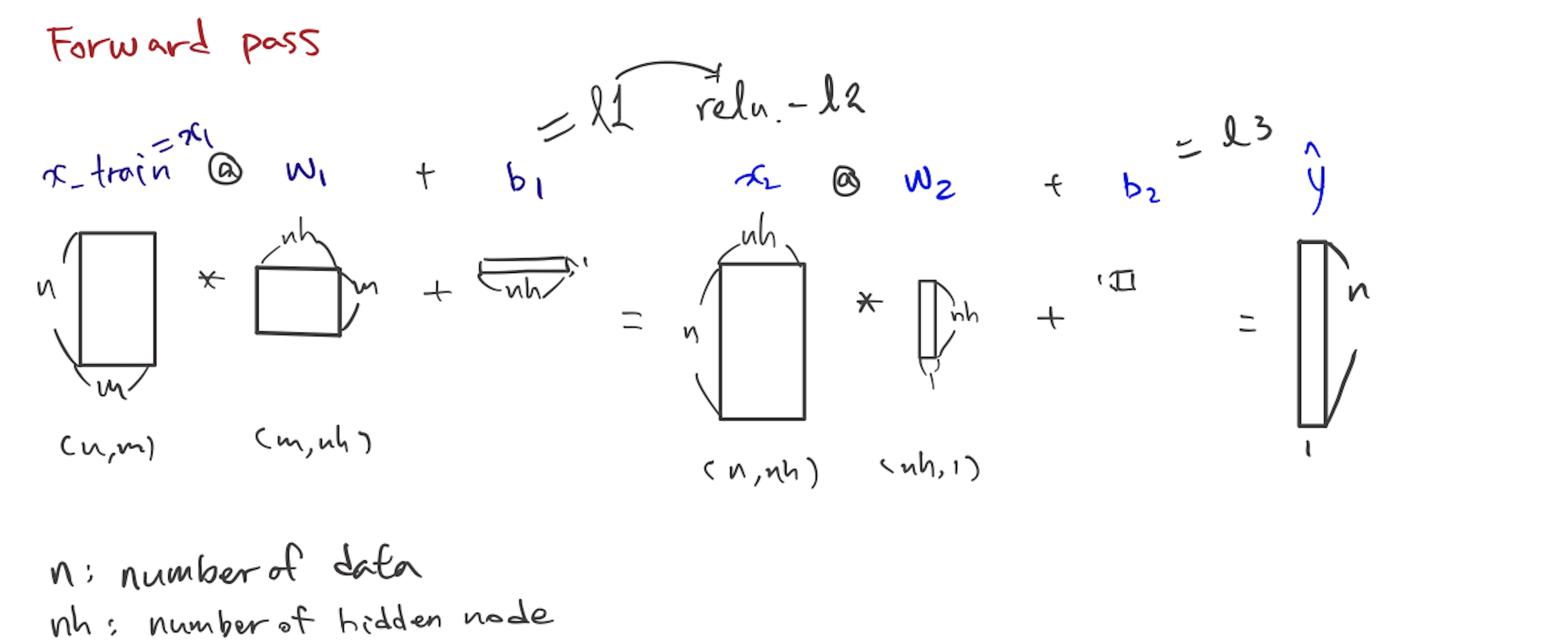### 2. Foundation version

• Gradients is output with respect to parameter
• we’ve done this work in this path(below)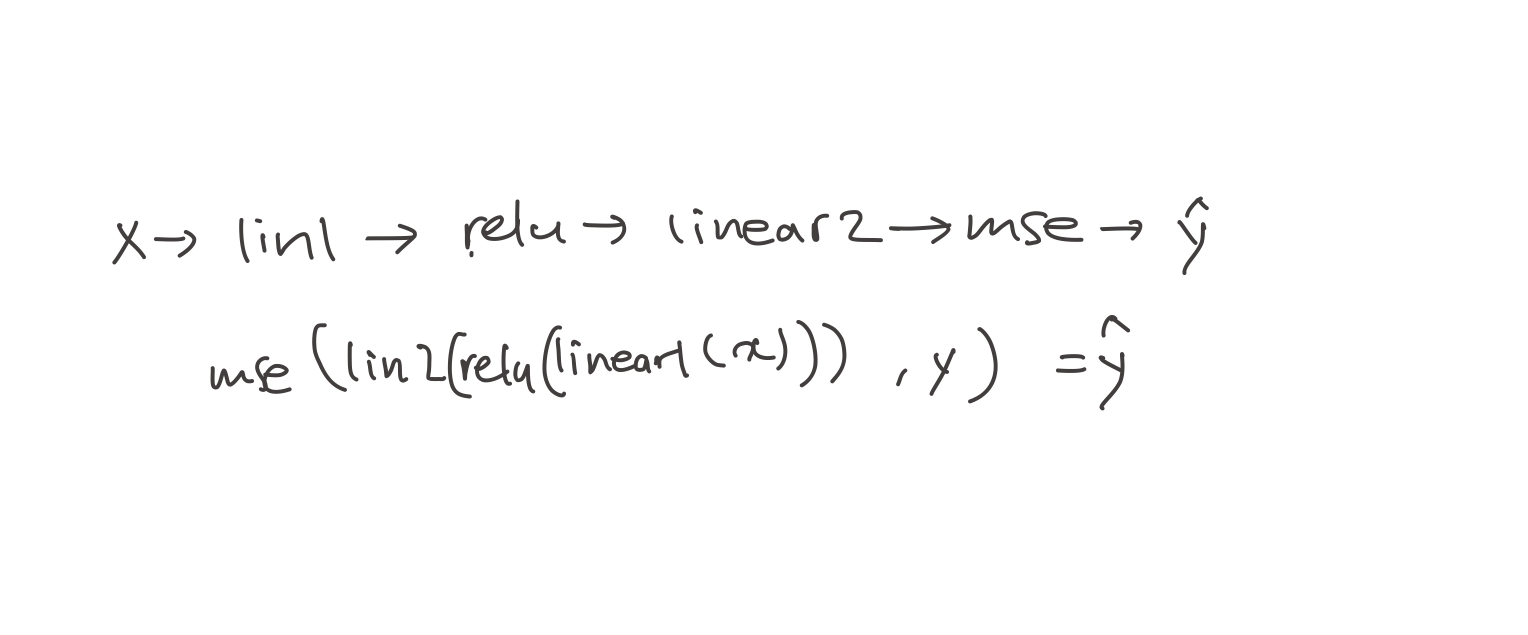• to simplify this calculus, we can just change it into

$y=f(u)$, $u=g(x)$

• So, you should know of the derivative of each bit on its own, and then you multiply them all together. As a result, it would be $dy$ over $dx$ cross over the data.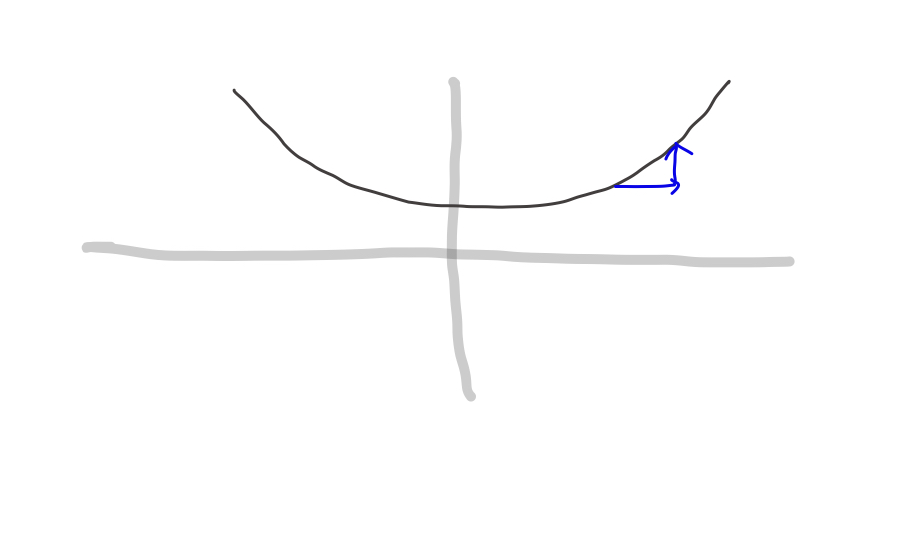• So you can get gradient, output with respect to parameter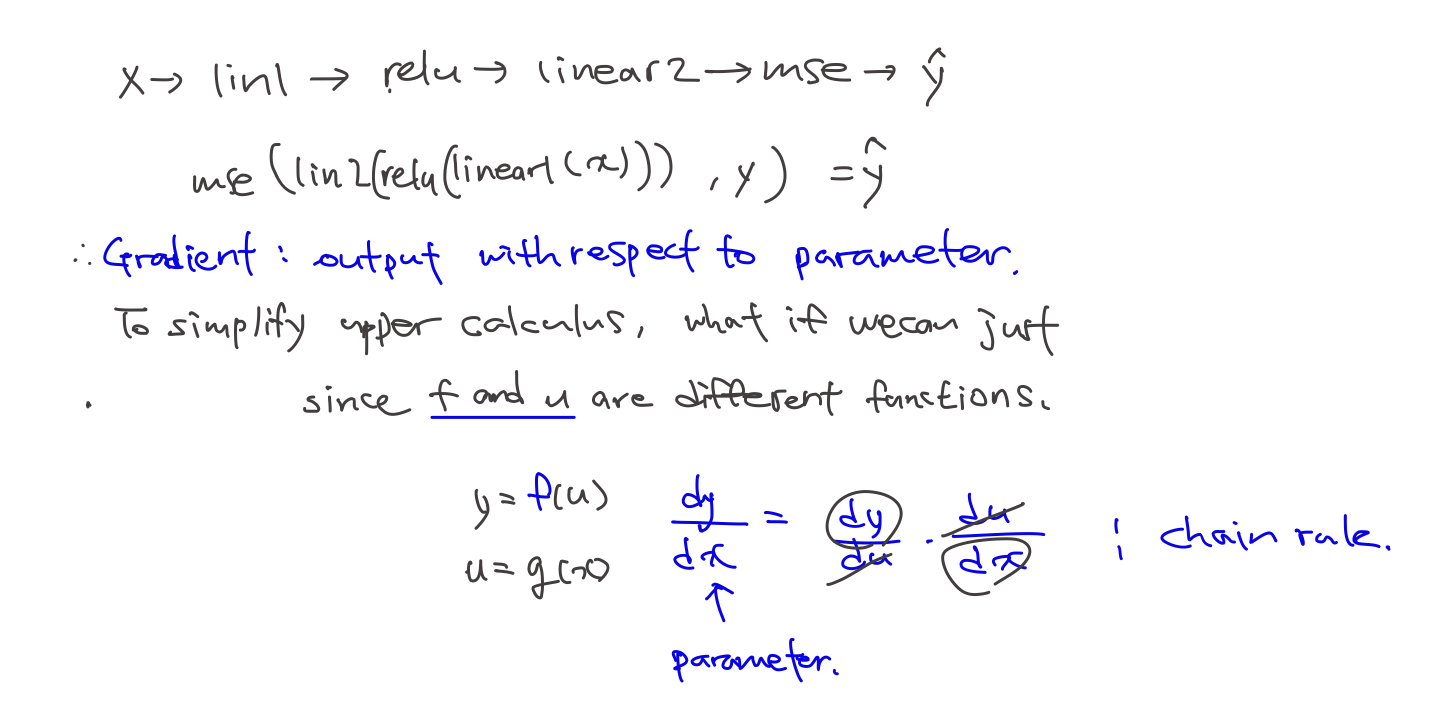• What order should we calculate?BTW, why Jeremy wrote $\hat y$, not Loss function?1

##### decompose function
• We want to get derivative of $w_1$ which forms $t=x_1 @ w_1 + b_1$
• But, we have a estimation of answer (we call it y hat) now
• So, I will decompose funciton to trace target variable.
• Using the above forward pass, we can suppose some function from the end.
• start from $\hat y$, We know MSE funciton got two parameters, output, $u$ and target $y$.
• from MSE’s input we know $linear_2$ function’s output and supposing v is input of that function, $linear_2(v) = u$
• similarly, v became output of $ReLU, ReLU(t) = v$##### chain rule with code
• examplify backward process by random sampling

• To get a variable, I modified forward model a little

def model_ping(out = 'x_train'):
l1 = lin(x_train, w1, b1) # one linear layer
l2 = relu(l1) # one relu layer
l3 = lin(l2, w2, b2) # one more linear layer
return eval(out)

• Be careful we don’t use mse_loss in backward process

1) start with the very last function, which is loss funciton. MSE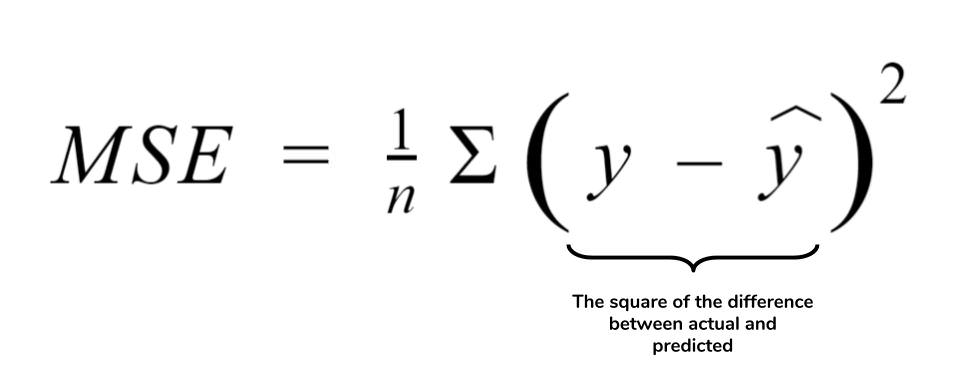• If we codify this formula,
def mse_grad(inp, targ):  #mse_input(1000,1), mse_targ (1000,1)
# grad of loss with respect to output of previous layer
inp.g = 2. * (inp.squeeze() - targ).unsqueeze(-1) / inp.shape

• And, this can be examplified like below.
• Notice that input of gradient function is same with forward function
y_hat = model_ping('l3') #get value from forward model
y_hat.g = ((y_hat.squeeze(-1)-y_train).unsqueeze(-1))/y_hat.shape

y_hat.g.shape
>>> torch.Size([50000, 1])

• We can just calculate using broadcasting, not using squeeze. then why should do and unsqueeze again?
🎯 It’s related with random access memory(RAM).. If I don’t squeeze, (I’m using colab) it out of RAM.

2) Derivative of linear2 function

• This process’s weight dimensions defined by axis=1, axis=2.
• axis=0 dimension means size of data. This will be summazed by .sum(0) method.
• unsqeeze(-1)&unsqeeze(1) seperates the dimension, and make a dot product, and vanish axis=0 dimension.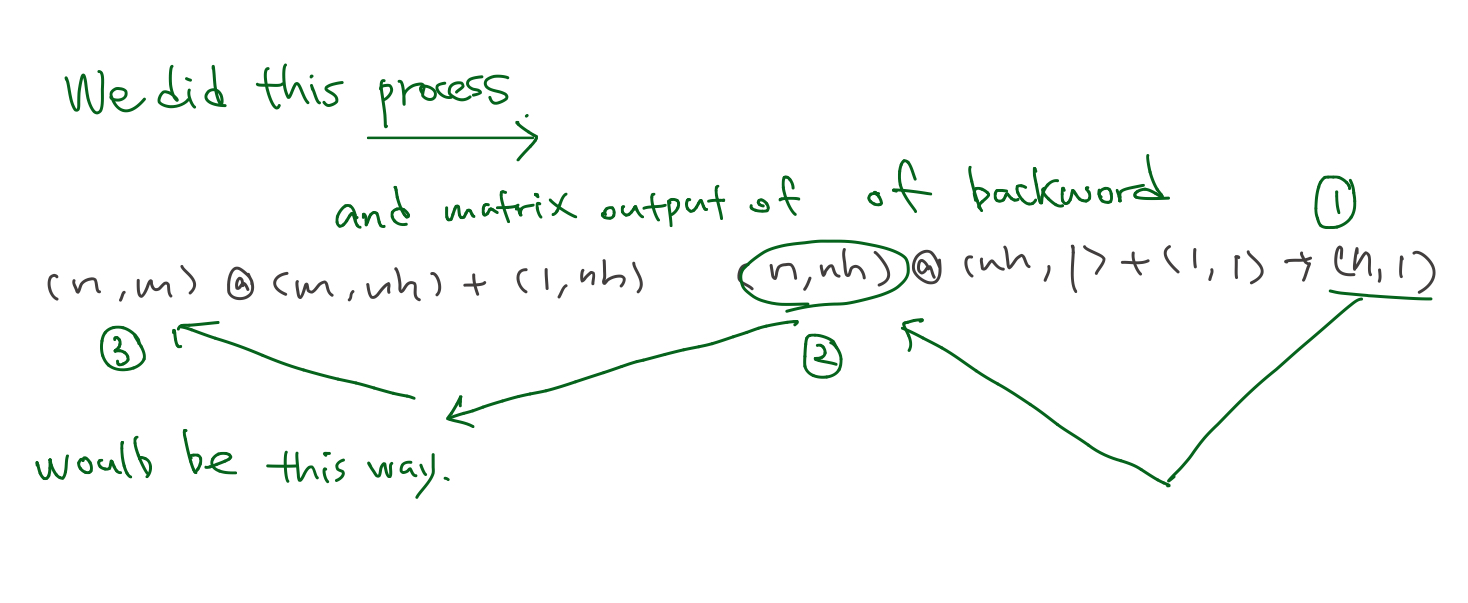def lin_grad(inp, out, w, b):
# grad of matmul with respect to input
inp.g = out.g @ w.t()
w.g = (inp.unsqueeze(-1) * out.g.unsqueeze(1)).sum(0)
b.g = out.g.sum(0)

• Examplified below
lin2 = model_ping('l2'); #get value from forward model
lin2.g = y_hat.g@w2.t();
w2.g = (lin2.unsqueeze(-1) * y_hat.g.unsqueeze(1)).sum(0);
b2.g = y_hat.g.sum(0);

lin2.g.shape, w2.g.shape, b2.g.shape
>>> torch.Size([50000, 50])torch.Size([50, 1])torch.Size()

• Notice going reverse order, we’re passing in gradient backward

3) derivative of ReLU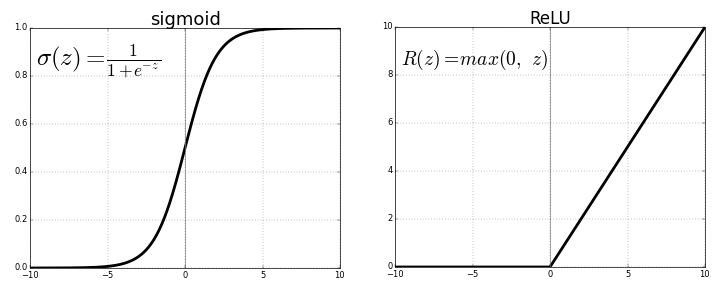def relu_grad(inp, out):
# grad of relu with respect to input activations
inp.g = (inp>0).float() * out.g

• Examplified below
lin1=model_ping('l1') #get value from forward model
lin1.g = (lin1>0).float() * lin2.g;
lin1.g.shape
>>> torch.Size([50000, 50])


4) Derivative of linear1

• Same process with 2) but, this process’s weight has
def lin_grad(inp, out, w, b):
# grad of matmul with respect to input
inp.g = out.g @ w.t()
w.g = (inp.unsqueeze(-1) * out.g.unsqueeze(1)).sum(0)
b.g = out.g.sum(0)

• Examplified below
x_train.g = lin1.g @ w1.t();
w1.g = (x_train.unsqueeze(-1) * lin1.g.unsqueeze(1)).sum(0);
b1.g = lin1.g.sum(0);

x_train.g.shape, w1.g.shape, b1.g.shape
>>> torch.Size([50000, 784])torch.Size([784, 50])torch.Size()


5) Then it goes backward pass

def forward_and_backward(inp, targ):
# forward pass:
l1 = inp @ w1 + b1
l2 = relu(l1)
out = l2 @ w2 + b2
# we don't actually need the loss in backward!
loss = mse(out, targ)

# backward pass:


Version 1 (Basic)- Wall time: 1.95 s

Summary

• Notice that output of function at forward pass became input of backward pass
• backpropagation is just the chain rule
• value loss (loss=mse(out,targ)) is not used in gradient calcuation.
• Because, it doesn’t appear with the weight.
• w1g, w2g, b1g, b2g, ig will be used for optimizer
##### check the result using Pytorch autograd
• require_grad_ is the magical function, which can automatic differentiation.2
• This magical auto gradified tensor keep track what happend in forward (taking loss function),
• and do the backward3
• So it saves our time to differentiate ourselves

⤵️ THis is benchmark…..

Version 2 (torch autograd)- Wall time: 3.81 µs

### 3. Refactor model

• Amazingly, just refactoring our main pieces, it comes down up to Pytorch package.

🌟 Implement yourself, Practice, practice, practice! 🌟

#### 3.1 Layers as classes

• Relu and Linear are layers in oue neural net. -> make it as classes

• For the forward, using __call__ for the both of forward & backward. Because ‘call’ means we treat this as a function.

class Lin():
def __init__(self, w, b): self.w,self.b = w,b

def __call__(self, inp):
self.inp = inp
self.out = inp@self.w + self.b
return self.out

def backward(self):
self.inp.g = self.out.g @ self.w.t()
# Creating a giant outer product, just to sum it, is inefficient!
self.w.g = (self.inp.unsqueeze(-1) * self.out.g.unsqueeze(1)).sum(0)
self.b.g = self.out.g.sum(0)

• Remember that in lin_grad function, we save bias&weight!!!!!

💬 inp.g : gradient of the output with respect to the input. {: style=”color:grey; font-size: 90%; text-align: center;”}
💬 w.g : gradient of the output with respect to the weight. {: style=”color:grey; font-size: 90%; text-align: center;”}
💬 b.g : gradient of the output with respect to the bias. {: style=”color:grey; font-size: 90%; text-align: center;”}

class Model():
def __init__(self, w1, b1, w2, b2):
self.layers = [Lin(w1,b1), Relu(), Lin(w2,b2)]
self.loss = Mse()

def __call__(self, x, targ):
for l in self.layers: x = l(x)
return self.loss(x, targ)

def backward(self):
self.loss.backward()
for l in reversed(self.layers): l.backward()

• refer to Jeremy’s Model class, he put layers in list

• Dionne’s self-study note: Decomposing Jeremy’s Model class
1. init needs weight, bias but not x data
2. when call that class(a.k.a function) it gave x data and y label!
3. jeremy composited function in layers. x = l(x) so concise…..
4. also utilized that layer list when backward ust reversing it (using python list’s method)
• And he is recursively calling the function on the result of the previous thing. ⬇️
for l in self.layers:
x = l(x)


Q2: Don’t I need to declare magical autograd function, requires_grad_?{: style=”color:red; font-size: 130%; text-align: center;”}

Version 3 (refactoring - layer to class)- Wall time: 5.25 µs

#### 3.2 Modue.forward()

1. Duplicate code makes execution time slow.
• Role of __call__ changed. No more __call__ for implementing forward pass.
• By initializing the forward with __call__, Module.forward() use overriding to maximize reusability. So any layer inherit Module, can use parent’s function.
2. gradient of the output with respect to the weight
(self.inp.unsqueeze(-1) * self.out.g.unsqueeze(1)).sum(0)


can be reexpressed using einsum,

torch.einsum("bi,bj->ij", inp, out.g)

• Defining forward and Module enables Pytorch to out almost duplicates

Version 4 (Module & einsum)- Wall time: 4.29 µs

Q2: Isn’t there any way to use broadcasting? Why we should use outer product?{: style=”color:red; font-size: 130%; text-align: center;”}

#### 3.3 Without einsum

Replacing einsum to matrix product is even more faster.

torch.einsum("bi,bj->ij", inp, out.g)


can be reexpressed using matrix product,

inp.t() @ out.g


Version 5 (without einsum)- Wall time: 3.81 µs

#### 3.4 nn.Linear and nn.Module

Torch’s package nn.Linear and nn.Module

Version 6 (torch package)- Wall time: 5.01 µs

• Final, Using torch.nn.Linear & torch.nn.Module ~~~python

class Model(nn.Module): def init(self, n_in, nh, n_out): super().init() self.layers = [nn.Linear(n_in,nh), nn.ReLU(), nn.Linear(nh,n_out)] self.loss = mse

def __call__(self, x, targ):
for l in self.layers: x = l(x)
return self.loss(x.squeeze(), targ)


class Model(): def init(self): self.layers = [Lin(w1,b1), Relu(), Lin(w2,b2)] self.loss = Mse()

def __call__(self, x, targ):
for l in self.layers: x = l(x)
return self.loss(x, targ)

def backward(self):
self.loss.backward()
for l in reversed(self.layers): l.backward()
`

~~~